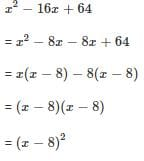Test: Factorisation

# Test: Factorisation

Test Description

## 10 Questions MCQ Test Mathematics (Maths) Class 9 | Test: Factorisation

Test: Factorisation for Class 9 2023 is part of Mathematics (Maths) Class 9 preparation. The Test: Factorisation questions and answers have been prepared according to the Class 9 exam syllabus.The Test: Factorisation MCQs are made for Class 9 2023 Exam. Find important definitions, questions, notes, meanings, examples, exercises, MCQs and online tests for Test: Factorisation below.
Solutions of Test: Factorisation questions in English are available as part of our Mathematics (Maths) Class 9 for Class 9 & Test: Factorisation solutions in Hindi for Mathematics (Maths) Class 9 course. Download more important topics, notes, lectures and mock test series for Class 9 Exam by signing up for free. Attempt Test: Factorisation | 10 questions in 10 minutes | Mock test for Class 9 preparation | Free important questions MCQ to study Mathematics (Maths) Class 9 for Class 9 Exam | Download free PDF with solutions
 1 Crore+ students have signed up on EduRev. Have you?
Test: Factorisation - Question 1

### Which of the following are the factors of a2 + ab +bc + ca

Test: Factorisation - Question 2

### Factoring 3x2-5x+2

Detailed Solution for Test: Factorisation - Question 2

3x^2-3x-2x+2
now, we take common
3x(x-1)-2(x-1)
(x-1)common and (3x-2)
(x-1)(3x-2)

Test: Factorisation - Question 3

### The number of zeros of x2 + 4x + 2

Test: Factorisation - Question 4

Factorise by splitting the middle term 2x- 11x + 12

Test: Factorisation - Question 5

The volume of cuboid is given by the expression x3+2x2-x-2. The dimension of the cuboid for x = 5 is:​

Test: Factorisation - Question 6

Expansion of 2x(x + 2y) + 3x(2x - 3y) yields

Test: Factorisation - Question 7

What are the two factors of quadratic polynomial x2-16x+64?

Detailed Solution for Test: Factorisation - Question 7Test: Factorisation - Question 8

Factorise the quadratic polynomial by splitting the middle term:
x2 + 14x + 45

Detailed Solution for Test: Factorisation - Question 8

The two numbers that add up to 14, and multiply to 45 and 9 and 5.

(x+5)(x+9)

Test: Factorisation - Question 9

Factorize the quadratic polynomial by splitting the middle term: y2 – 4 y –21​

Test: Factorisation - Question 10

What is the value of p if x-2 is a factor of x2 – 6x + p ?

Detailed Solution for Test: Factorisation - Question 10

P(x) = ×2-6×+p
p (2)= (2)2-6 (2) +p
4- 12 + p
-8 + p
p = -8

## Mathematics (Maths) Class 9

88 videos|397 docs|109 tests
 Use Code STAYHOME200 and get INR 200 additional OFF Use Coupon Code